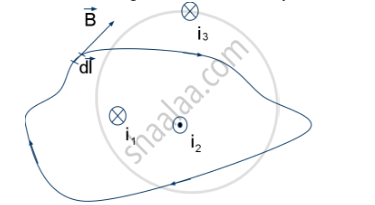# State Ampere'S Circuital Law, Expressing It in the Integral Form - Physics

State Ampere’s circuital law

State Ampere's circuital law, expressing it in the integral form

#### Solution 1

Ampere’s circuital law states that the line integral of magnetic field induction vecB  around a closed path in vacuum is equal to μ0 times the total current I passing through the surface, i.e. ointvecB.vec(dl)=mu_0I

#### Solution 2

Ampere’s Circuital Law states that the circulation of the resultant magnetic field along a closed, plane curve is equal to μo times the total current crossing the area bounded by the closed curve, provided the electric field inside the loop remains constant.In the above illustration, the Ampere’s Circuital Law can be written as follows:

ointvecB.vec(dl)=mu_0i

where, i=|i1i2|

Is there an error in this question or solution?
2014-2015 (March) Delhi Set 2

Share